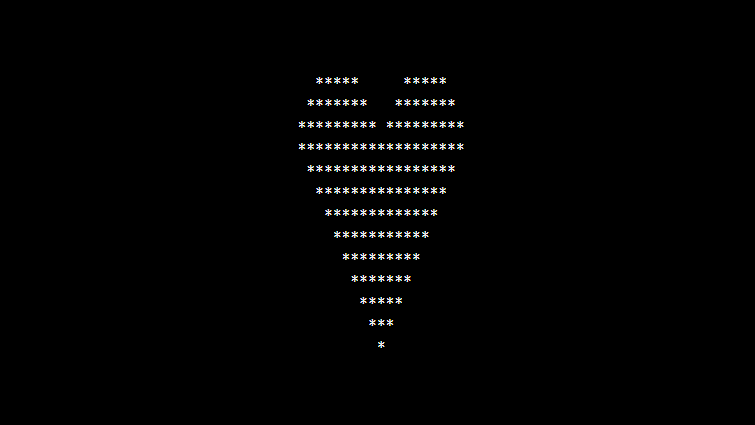# PHP program to print Heart Star Pattern

In this article write a PHP program to print Heart Star Pattern using asterisk (*) symbol. We run for loop 10 times, in which you have two outer for loops which contains nested for loops to print Heart Star Pattern of stars as shown in the following program.

## PHP program to print Heart Star Pattern

```<?php
echo "<pre>";
\$num = 10;
for(\$i=\$num/2; \$i<=\$num; \$i+=2)
{
for(\$j=1; \$j<\$num-\$i; \$j+=2)
{
echo "&nbsp;";
}
for(\$j=1; \$j<=\$i; \$j++)
{
echo "*";
}
for(\$j=1; \$j<=\$num-\$i; \$j++)
{
echo "&nbsp;";
}
for(\$j=1; \$j<=\$i; \$j++)
{
echo "*";
}
echo "<br/>";
}

for(\$i=\$num; \$i>=1; \$i--)
{
for(\$j=\$i; \$j<\$num; \$j++)
{
echo "&nbsp;";
}
for(\$j=1; \$j<=(\$i*2)-1; \$j++)
{
echo "*";
}
echo "<br/>";
}
echo "</pre>";
?>
```

### Output: